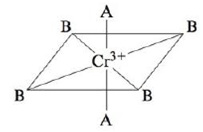# Consider the pseudo-octahedral complex ion of Cr 3+ , where A and B represent ligands. Ligand A produces a stronger crystal field than ligand B. Draw an appropriate crystal field diagram for this complex ion (assume the A ligands are on the z-axis).### Chemistry: An Atoms First Approach

2nd Edition
Steven S. Zumdahl + 1 other
Publisher: Cengage Learning
ISBN: 9781305079243

#### Solutions

Chapter
Section### Chemistry: An Atoms First Approach

2nd Edition
Steven S. Zumdahl + 1 other
Publisher: Cengage Learning
ISBN: 9781305079243
Chapter 20, Problem 90CP
Textbook Problem
1 views

## Consider the pseudo-octahedral complex ion of Cr3+, where A and B represent ligands.Ligand A produces a stronger crystal field than ligand B. Draw an appropriate crystal field diagram for this complex ion (assume the A ligands are on the z-axis).

Interpretation Introduction

Interpretation: An appropriate crystal field diagram for the given complex ion of Cr+3 is to be drawn. Ligand A is considered to be a strong crystal field ligand and it is on the z axis.

Concept introduction: The electrons in the d orbital of a transition metal split into high and low energy orbitals when ligands are attached to it. The energy difference between these two levels depends upon the properties of both metal and the ligands. If the ligand is strong, then splitting will be high and the complex will be low spin. If the ligand is weak, then splitting will be less and the complex will be high spin.

To determine: An appropriate crystal field diagram for the given complex ion of Cr+3 .

### Explanation of Solution

Explanation

The given complex is,

Figure 1

The central metal is Cr and charge on it is +3 .

The atomic number of Cr is 24 , hence its electronic configuration is [Ar]3d54s1 . With the loss of three electrons, it becomes Cr+3 and its electronic configuration is [Ar]3d3

### Still sussing out bartleby?

Check out a sample textbook solution.

See a sample solution

#### The Solution to Your Study Problems

Bartleby provides explanations to thousands of textbook problems written by our experts, many with advanced degrees!

Get Started

Find more solutions based on key concepts
What wind system flows along the northern margin?

Fundamentals of Physical Geography

Eating disorders are common only in cultures where extreme thinness is an ideal. Who in society do you think se...

Nutrition: Concepts and Controversies - Standalone book (MindTap Course List)

How can astronomers detect neutrinos from the sun?

Horizons: Exploring the Universe (MindTap Course List)

Draw an ocean wave and label its parts. Include a definition of wave period.

Oceanography: An Invitation To Marine Science, Loose-leaf Versin

An aluminum wire having a cross-sectional area equal to 4.00 106 m2 carries a current of 5.00 A. The density o...

Physics for Scientists and Engineers, Technology Update (No access codes included)